# Pascal’s Triangle – Binomial Theorem

• Difficulty Level : Basic
• Last Updated : 30 Apr, 2021

We know the expansions of terms like (x + 2) and (x + 3)3. These are pretty simple to do, but sometimes we come across some expressions like (x+2)5. Expressions like these are hard to expand, we can use some tricks to simplify for example,

(x+2)5 = (x+2)(x + 2)2(x+2)2

Researcher work on the binomial expansions which involve thousands of exponents. It becomes essential to know a simple way to expand them. This is still a lot of work, that’s where binomial theorem comes to our rescue. It allows us to expand any general expressions like (x+ a)n. Let’s look at this theorem in detail.

### Binomial Expression

A binomial expression is defined as an expression that has two terms that are connected by operators like + or -.  For example, x + a, x – 6, and so on are examples of binomial expressions. Raising a binomial expression to a power greater than 3 is pretty hard and cumbersome. Let’s see some binomial expansions and try to find some pattern in them,

(x + a)0 =                                                                                                              1

(x + a)1 =                                                                                                           x + a

(x + a)2 =                                                                                                    x2 + 2ax + a2

(x + a)3 =                                                                                             x3 + 3a2x + 3ax2 + a3

Notice, we need to know the coefficients of these terms, and then it can be a little easier for us to describe their expansions. Pascals’ triangle can be used to generate these results really quickly.

### What is Pascal’s Triangle?

It is named after the famous Philosopher and Mathematician ‘Pascal’ who developed a pattern of numbers starting with 1 and the numbers beneath are the summation of the above numbers. To start making Pascal’s triangle, first, write down number 1. The second row is written down by two 1s again. Other rows are generated using the previous rows to make a triangle of numbers. Each row begins and ends with a 1.

1
1           1
1          x          1
1        x         x          1

The numbers that are given by x are calculated by adding the numbers from the previous row, which lie on the left and right above the given position. The figure below demonstrates the process of building Pascal’s triangle.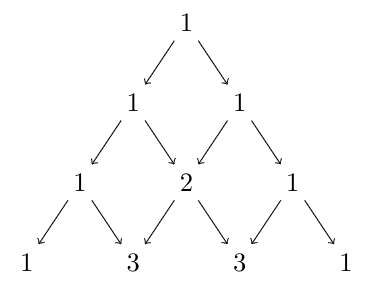In this way, Pascal’s triangle can be generated.

Question 1: Generate the sixth row of Pascal’s triangle.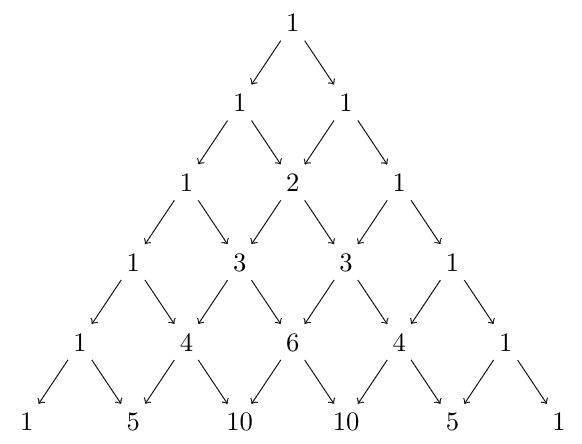Question 2: Generate the tenth row of Pascal’s triangle.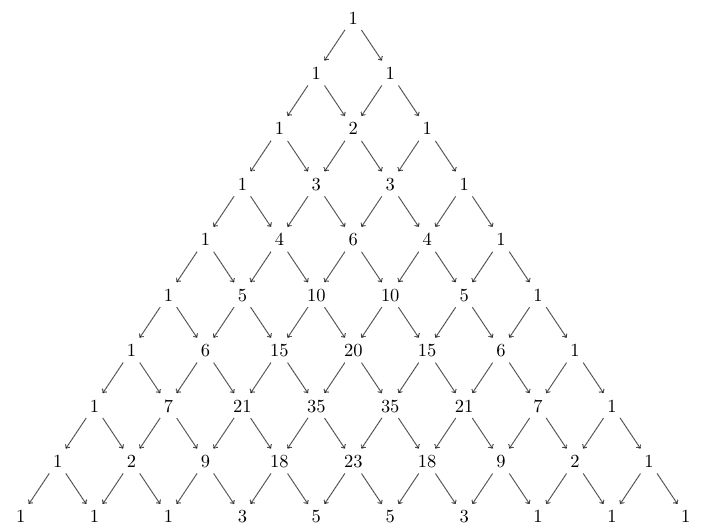### Pascal’s Triangles for expanding Binomial Expressions

Let’s see the above equations again,

(x + a)0 =                                                                                1

(x + a)1 =                                                                             x + a

(x + a)2 =                                                                      x2 + 2ax + a2

(x + a)3 =                                                               x3 + 3a2x + 3ax2 + a3

We can conclude a few things from these equations for (x + a)n

1. There’s always one more term than the value of n.
2. For each term, the sum of exponents is always equal to n.
3. For the variable “x”, the exponent starts from n and keeps on decreasing till zero. Similarly, for “a” exponent starts from 0 and goes till n.
4. Coefficients of these terms are given by Pascal’s triangle.

Let’s see an example, suppose we want to expand (x + a)3 through this expansion concept. There should be four terms and the terms should have a decreasing exponent of “x” and an increasing exponent of “a” respectively.

(x + a)3 = C1x3 + C2x2a + C3xa2 + C4a3

The values C1, C2, C3, and C4 are coefficients, we will figure out the coefficients with Pascal’s triangle. Let’s see Pascal’s triangle with n + 1 rows,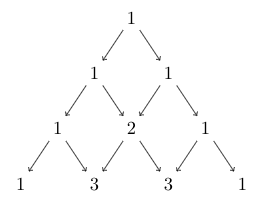The values in the last row give us the value of coefficients C1, C2, C3, and C4

Here, C1 = 1, C2 = 3, C3 = 3 and C4 = 1.

So, in this way, we can expand our binomial expressions.

For any binomial expression, (x + a)n the expansions is given by,

(a + b)n = c0anb0 + c1an-1b1 + c2an-2b2 + …. + cn-1a1bn-1 + cna0bn

The coefficients are given by the n+1 row of the Pascal’s triangle.

### Sample Problems

Question 1: Expand and verify (a + b)2

Solution:

First write the generic expressions without the coefficients.

(a + b)2 = c0a2b0 + c1a1b1 + c2a0b2

Now let’s build a Pascal’s triangle for 3 rows to find out the coefficients.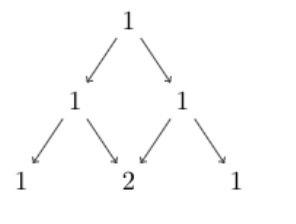The values of the last row give us the value of coefficients.

c0 = 1, c1 = 2, c2 =1

(a + b)2 = a2b0 + 2a1b1 + a0b2

Thus verified.

Question 2: Expand (a + b)4

Solution:

First write the generic expressions without the coefficients.

(a + b)4 = c0a4b0 + c1a3b1 + c2a2b2 + c3a1b3 + c4a0b4

Now let’s build a Pascal’s triangle for 5 rows to find out the coefficients.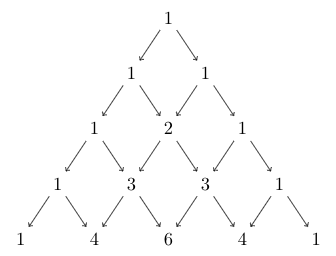The values of the last row give us the value of coefficients.

c0 = 1, c1 = 4, c2 = 6, c3 = 4 and c4 =1.

Thus, (a + b)4 = a4b0 + 4a3b1 +6a2b2 + 4a1b3 + a0b4

Question 3: Expand (a + b)5

Solution:

First write the generic expressions without the coefficients.

(a + b)5 = c0a5b0 + c1a4b1 + c2a3b2 + c3a2b3 + c4a1b4 + c5a0b5

Now let’s build a Pascal’s triangle for 6 rows to find out the coefficients.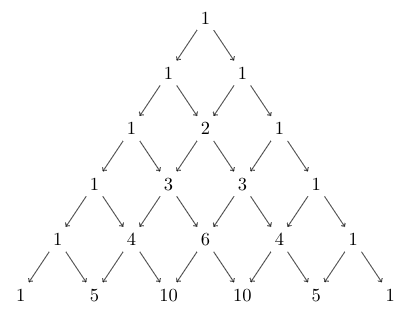The values of the last row give us the value of coefficients.

c0 = 1, c1 = 5, c2 = 10, c3 = 10, c4 =5 and c5 = 1.

(a + b)5 = a5b0 + 5a4b1 + 10a3b2 + 10a2b3 + 5a1b4 + a0b5

Question 4: Expand (a + b)6

Solution:

First write the generic expressions without the coefficients.

(a + b)6 = c0a6b0 + c1a5b1 + c2a4b2 + c3a3b3 + c4a2b4 + c5a1b5 + c6a0b

Now let’s build a Pascal’s triangle for 7 rows to find out the coefficients.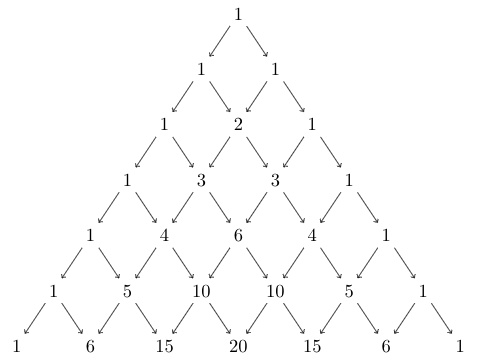The values of the last row give us the value of coefficients.

c0 = 1, c1 = 6, c2 = 15, c3 = 20, c4 =15, c5 = 6 and c6 = 1.

(a + b)6 = 1a6b0 + 6a5b1 + 15a4b2 + 20a3b3 + 15a2b4 + 6a1b5 + 1a0b

My Personal Notes arrow_drop_up